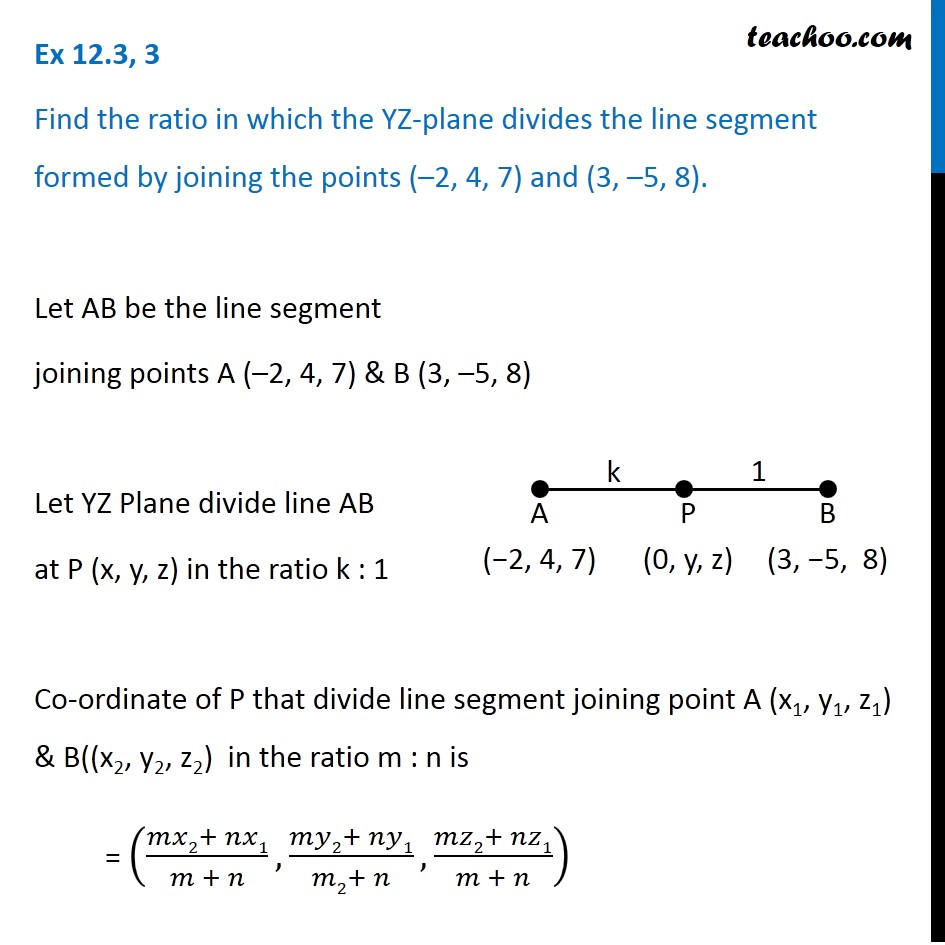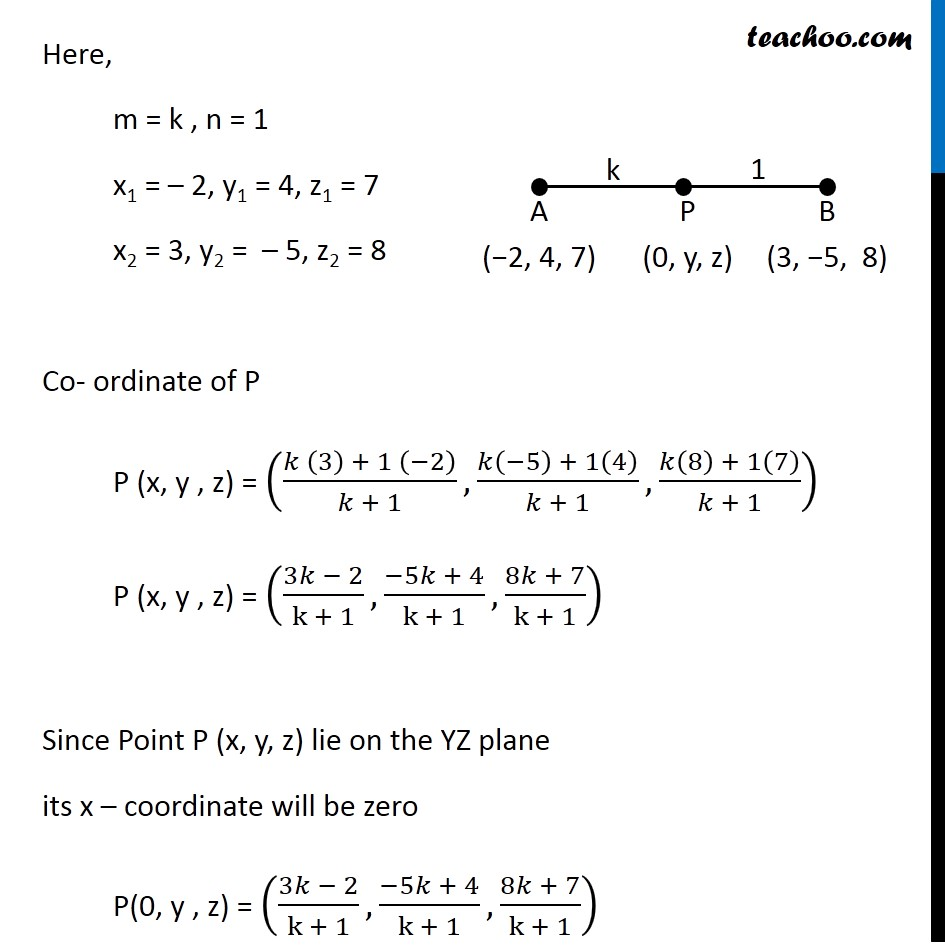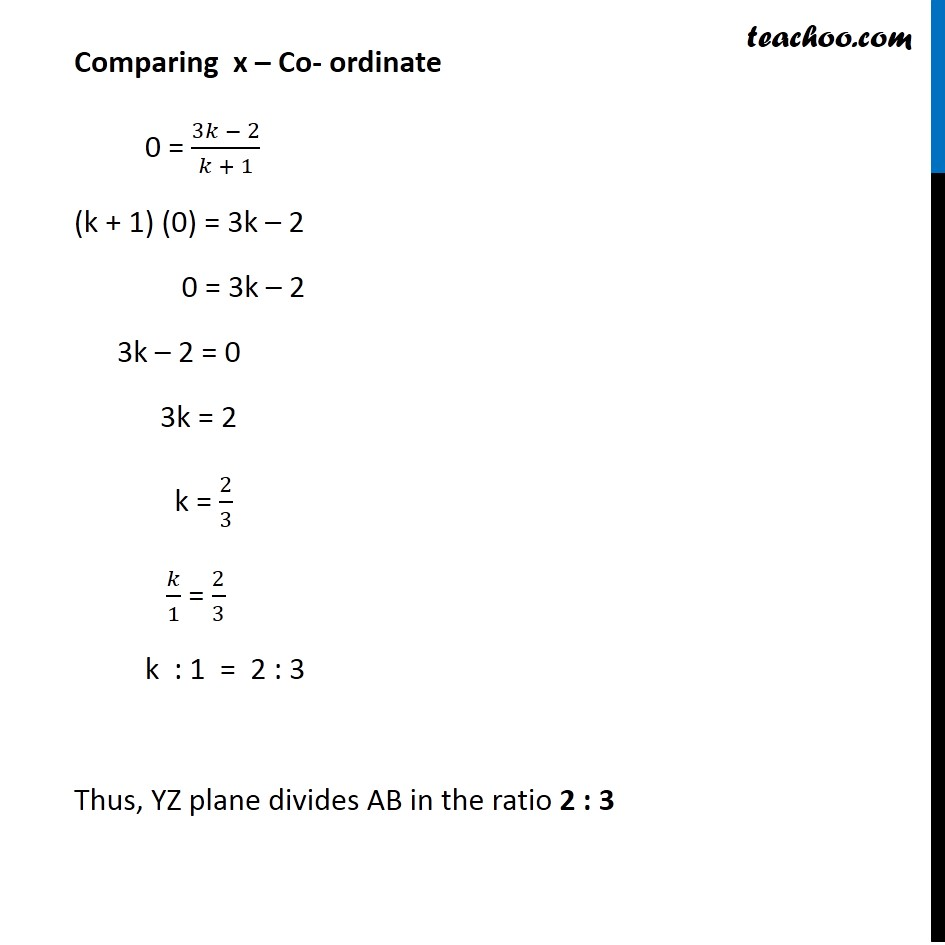Section Formula in 3D Geometry

Chapter 11 Class 11 - Intro to Three Dimensional Geometry
Serial order wiseLearn in your speed, with individual attention - Teachoo Maths 1-on-1 Class

### Transcript

Question 3 Find the ratio in which the YZ-plane divides the line segment formed by joining the points (–2, 4, 7) and (3, –5, 8). Let AB be the line segment joining points A (–2, 4, 7) & B (3, –5, 8) Let YZ Plane divide line AB at P (x, y, z) in the ratio k : 1 Co-ordinate of P that divide line segment joining point A (x1, y1, z1) & B((x2, y2, z2) in the ratio m : n is = ((𝑚𝑥2+ 𝑛𝑥1)/(𝑚 + 𝑛), (𝑚𝑦2+ 𝑛𝑦1)/(𝑚2+ 𝑛),(𝑚𝑧2+ 𝑛𝑧1)/(𝑚 + 𝑛)) Here, m = k , n = 1 x1 = – 2, y1 = 4, z1 = 7 x2 = 3, y2 = – 5, z2 = 8 Co- ordinate of P P (x, y , z) = ((𝑘 (3) + 1 (−2))/(𝑘 + 1), (𝑘(−5) + 1(4))/(𝑘 + 1), (𝑘(8) + 1(7))/(𝑘 + 1)) P (x, y , z) = ((3𝑘 − 2)/(k + 1),(−5𝑘 + 4)/(k + 1),(8𝑘 + 7)/(k + 1)) Since Point P (x, y, z) lie on the YZ plane its x – coordinate will be zero P(0, y , z) = ((3𝑘 − 2)/(k + 1),(−5𝑘 + 4)/(k + 1),(8𝑘 + 7)/(k + 1)) Comparing x – Co- ordinate 0 = (3𝑘 − 2)/(𝑘 + 1) (k + 1) (0) = 3k – 2 0 = 3k – 2 3k – 2 = 0 3k = 2 k = 2/3 𝑘/1 = 2/3 k : 1 = 2 : 3 Thus, YZ plane divides AB in the ratio 2 : 3